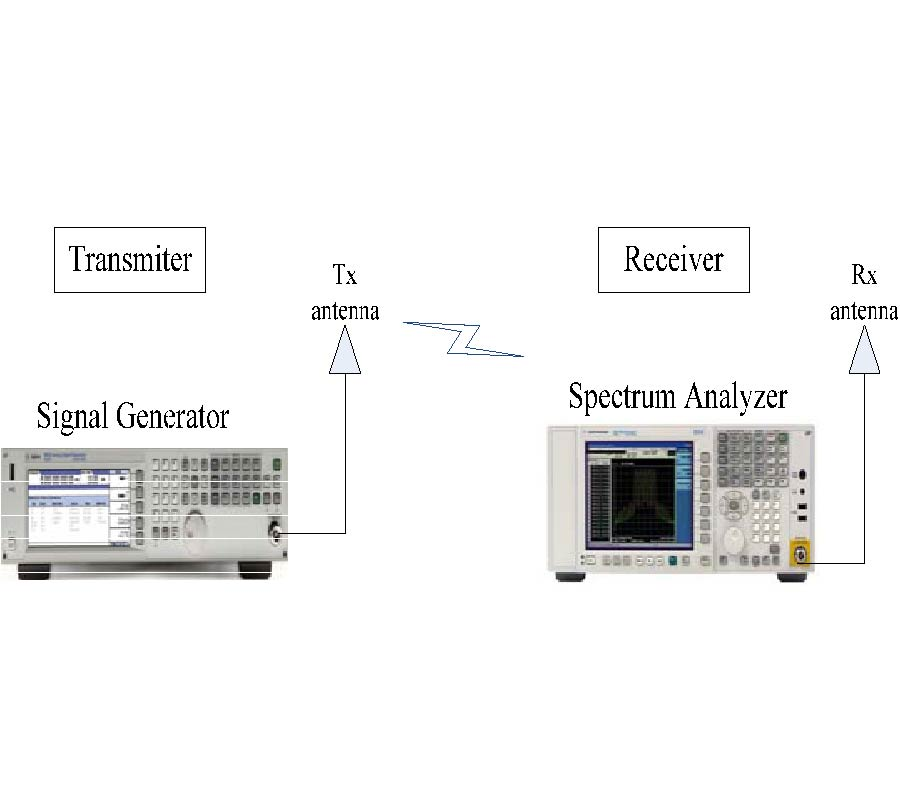# Vol. 56

Front:[PDF file] Back:[PDF file]
Latest Volume
All Volumes
All Issues
2017-05-09

#### Horizontal Diffraction in Multiple Obstacles Using Parabolic Equation with Recursive Convolution Nonlocal Boundary Conditions

By Zan-Yu Ge, Guizhen Lu, Huai-Bao Xiao, Dongdong Zeng, and Abomakhleb Gheit
Progress In Electromagnetics Research M, Vol. 56, 179-187, 2017
doi:10.2528/PIERM17031507

## Abstract

The accuracy of wave propagation prediction is very important in telecommunication network planning. The parabolic equation model has an advantage in computation efficiency and accuracy for wave propagation prediction. The recursive convolution nonlocal boundary condition has an advantage in improving the computational efficiency. In this paper, the recursive convolution nonlocal boundary conditions are extended to deal with the issue of horizontal diffraction loss in multiple obstacles. The validation is performed with experiments and the results show a good agreement.

## Citation

Zan-Yu Ge, Guizhen Lu, Huai-Bao Xiao, Dongdong Zeng, and Abomakhleb Gheit, "Horizontal Diffraction in Multiple Obstacles Using Parabolic Equation with Recursive Convolution Nonlocal Boundary Conditions," Progress In Electromagnetics Research M, Vol. 56, 179-187, 2017.
doi:10.2528/PIERM17031507
http://www.jpier.org/PIERM/pier.php?paper=17031507

## References

1. Levy, M. F., Parabolic Equation Methods for Electromagnetic Wave Propagation, IEE Press, London, 2000.
doi:10.1049/PBEW045E

2. Donohue, D. J. and J. R. Kuttler, "Propagation model in over terrain using the parabolic wave equation," IEEE Transactions on Antennas and Propagation, Vol. 48, No. 1, 824-827, 2001.

3. Wang, K. and Y. L. Long, "Propagation modeling over irregular terrain by the improved two-way parabolic equation method," IEEE Transactions on Antennas and Propagation, Vol. 60, No. 9, 4467-4471, 2012.
doi:10.1109/TAP.2012.2207063

4. Huang, Z. X., Q. Wu, and X. L. Wu, "Solving multi-object radar cross section based on wide-angle parabolic equation method," Systems Engineering and Electronics, Vol. 17, No. 4, 722-724, 2006.
doi:10.1016/S1004-4132(07)60005-X

5. Chiou, M. M. and J. F. Kiang, "Simulation of X-band signals in a sand and dust storm with parabolic wave equation method and two-ray model," IEEE Antennas and Wireless Propagation Letters, No. 99, 2016.

6. Valtr, P., P. Pechac, V. Kvicera, and M. Grabner, "A radiowave propagation study in an urban environment using the fourier split-step parabolic equation," The Second European Conference on Antennas and Propagation, EuCAP 2007, 2007.

7. Aklilu, T. and G. Giorges, "Finite element and finite difference methods for elliptic and parabolic differential equations," Numerical Analysis - Theory and Application, Prof. Jan Awrejcewicz (Ed.), InTech, 2011, ISBN: 978-953-307-389-7.

8. Fadugba, S. E., O. H. Edogbanya, and S. C. Zelibe, "Crank Nicolson Method for solving parabolic partial differential equations," IJA2M, Vol. 1, No. 3, 8-23, 2013.

9. Silva, M. A. N., E. Costa, and M. Liniger, "Analysis of the effects of irregular terrain on radio wave propagation based on a three-dimensional parabolic equation," IEEE Transactions on Antennas and Propagation, Vol. 60, No. 4, 2138-2143, 2012.
doi:10.1109/TAP.2012.2186227

10. Sevgi, L., C. Uluisik, and F. Akleman, "A MATLAB-based two-dimensional parabolic equation radiowave propagation package," IEEE Antennas and Propagation Magazine, Vol. 47, No. 4, 164-175, 2005.
doi:10.1109/MAP.2005.1589923

11. Bai, R. J., C. Liao, N. Sheng, and Q. H. Zhang, "Prediction of wave propagation over digital terrain by parabolic equation model," IEEE International Symposium on MAPE, Oct. 29-31, 2013.

12. Keefe, K., "Dispersive waves and PML performance for large-stencil differencing in the parabolic equation," IEEE Transactions on Antennas and Propagation, Vol. 60, No. 11, 5268-5277, 2012.
doi:10.1109/TAP.2012.2208090

13. Zhang, P., L. Bai, Z. Wu, and F. Li, "Effect of window function on absorbing layers top boundary in parabolic equation," Antennas and Propagation (APCAP), Jul. 26-29, 2014.

14. Dalrymple, R. A. and P. A. Martin, "Perfect boundary conditions for parabolic water-wave models," Proc. R. Soc. London A, Vol. 437, 41-54, 1992.
doi:10.1098/rspa.1992.0045

15. Zebic-Le Hyaric, A., "Wide-angle nonlocal boundary conditions for the parabolic wave equation," IEEE Transactions on Antennas and Propagation, Vol. 49, No. 6, 916-922, 2001.
doi:10.1109/8.931149

16. Mias, C., "Fast computation of the nonlocal boundary condition in finite difference parabolic equation radiowave propagation simulations," IEEE Transactions on Antennas and Propagation, Vol. 56, No. 6, 1699-1705, 2008.
doi:10.1109/TAP.2008.923341

17. Claerbout, J. F., Fundamentals of Geophysical Data Processing with Application to Petroleum Prospect, McGraw-Hill Press, New York, 1976.

18. Mardeni, R. and K. F. Kwan, "Optimization of hata propagation prediction model in suburban area in Malaysia," Progress In Electromagnetics Research C, Vol. 13, 91-106, 2010.

19. Chang, W. and L. Tsang, "A partial coherent physical model of third and fourth Stokes parameters of Sastrugi snow surfaces over layered media with rough surface boundary conditions of conical scattering combined with vector radiative transfer theory," Progress In Electromagnetics Research B, Vol. 45, 57-82, 2012.
doi:10.2528/PIERB12081514

20. Ye, H. and Y. Q. Jin, "Parameterization of the tapered incident wave for numerical simulation of electromagnetic scattering from rough surface," IEEE Transactions on Antennas and Propagation, Vol. 53, No. 3, 1234-1237, 2005.
doi:10.1109/TAP.2004.842586